# Forward-Backward Splitting¶

$\newcommand{\dotp}{\langle #1, #2 \rangle}$ $\newcommand{\enscond}{\lbrace #1, #2 \rbrace}$ $\newcommand{\pd}{ \frac{ \partial #1}{\partial #2} }$ $\newcommand{\umin}{\underset{#1}{\min}\;}$ $\newcommand{\umax}{\underset{#1}{\max}\;}$ $\newcommand{\umin}{\underset{#1}{\min}\;}$ $\newcommand{\uargmin}{\underset{#1}{\arg\min}\;}$ $\newcommand{\norm}{\|#1\|}$ $\newcommand{\abs}{\left|#1\right|}$ $\newcommand{\choice}{ \left\{ \begin{array}{l} #1 \end{array} \right. }$ $\newcommand{\pa}{\left(#1\right)}$ $\newcommand{\diag}{{diag}\left( #1 \right)}$ $\newcommand{\qandq}{\quad\text{and}\quad}$ $\newcommand{\qwhereq}{\quad\text{where}\quad}$ $\newcommand{\qifq}{ \quad \text{if} \quad }$ $\newcommand{\qarrq}{ \quad \Longrightarrow \quad }$ $\newcommand{\ZZ}{\mathbb{Z}}$ $\newcommand{\CC}{\mathbb{C}}$ $\newcommand{\RR}{\mathbb{R}}$ $\newcommand{\EE}{\mathbb{E}}$ $\newcommand{\Zz}{\mathcal{Z}}$ $\newcommand{\Ww}{\mathcal{W}}$ $\newcommand{\Vv}{\mathcal{V}}$ $\newcommand{\Nn}{\mathcal{N}}$ $\newcommand{\NN}{\mathcal{N}}$ $\newcommand{\Hh}{\mathcal{H}}$ $\newcommand{\Bb}{\mathcal{B}}$ $\newcommand{\Ee}{\mathcal{E}}$ $\newcommand{\Cc}{\mathcal{C}}$ $\newcommand{\Gg}{\mathcal{G}}$ $\newcommand{\Ss}{\mathcal{S}}$ $\newcommand{\Pp}{\mathcal{P}}$ $\newcommand{\Ff}{\mathcal{F}}$ $\newcommand{\Xx}{\mathcal{X}}$ $\newcommand{\Mm}{\mathcal{M}}$ $\newcommand{\Ii}{\mathcal{I}}$ $\newcommand{\Dd}{\mathcal{D}}$ $\newcommand{\Ll}{\mathcal{L}}$ $\newcommand{\Tt}{\mathcal{T}}$ $\newcommand{\si}{\sigma}$ $\newcommand{\al}{\alpha}$ $\newcommand{\la}{\lambda}$ $\newcommand{\ga}{\gamma}$ $\newcommand{\Ga}{\Gamma}$ $\newcommand{\La}{\Lambda}$ $\newcommand{\si}{\sigma}$ $\newcommand{\Si}{\Sigma}$ $\newcommand{\be}{\beta}$ $\newcommand{\de}{\delta}$ $\newcommand{\De}{\Delta}$ $\newcommand{\phi}{\varphi}$ $\newcommand{\th}{\theta}$ $\newcommand{\om}{\omega}$ $\newcommand{\Om}{\Omega}$

In :
from __future__ import division
%pylab inline

Populating the interactive namespace from numpy and matplotlib


This numerical tour presents the Forward-Backward (FB) algorithm to minimize the sum of a smooth and a simple function. It shows an application to sparse deconvolution.

## Forward-Backward Algorithm¶

We consider the problem of minimizing the sum of two functions $$E^\star = \umin{x \in \RR^N} E(x) = f(x) + g(x).$$

So, we want to find a vector $x^\star$ solution to the problem, i.e. a minimizer of $E=f+g$.

We assume that $f$ is a $C^1$ function with $\beta$-Lipschitz gradient.

We also assume that $g$ is "simple", in the sense that one can compute exactly and quickly its proximity operator, which is defined as $$\text{prox}_{\ga g}(x) = \uargmin{y \in \RR^N} \frac{1}{2}\norm{x-y}^2 + \ga g(y).$$ for any $\ga > 0$.

The forward-backward algorithm reads, after initializing $x^{(0)} \in \RR^N$, $$x^{(k+1)} = \text{prox}_{\ga g}\pa{ x^{(k)} - \ga \nabla f( x^{(k)} ) }.$$

If $0 < \ga < 2/\beta$, then this scheme converges to a minimizer of $f+g$.

## Sparse Regularization of Inverse Problems¶

We consider a linear inverse problem $$y = A x^\sharp + w \in \RR^P$$ where $x^\sharp \in \RR^N$ is the (unknown) signal to recover, $w \in \RR^P$ is a noise vector, and $A \in \RR^{P \times N}$ models the acquisition device.

To recover an estimate of the signal $x^\sharp$, we consider basis pursuit denoising, which makes use of the $\ell^1$ norm as sparsity enforcing penalty: $$\umin{x \in \RR^N} \frac{1}{2} \norm{A x-y}^2 + \la \norm{x}_1,$$ where the $\ell^1$ norm is defined as $$\norm{x}_1 = \sum_i \abs{x_i}.$$

The parameter $\la$ should be set in accordance to the noise level $\norm{w}$.

This minimization problem can be cast in the form of minimizing $f+g$ where $$f(x) = \frac{1}{2} \norm{Ax-y}^2 \qandq g(x) = \la \norm{x}_1.$$

$f$ is smooth; we have $$\nabla f(x) = A^* (A x - y),$$ which is $\beta$-Lipschitz continuous, with $$\beta = \norm{ A^* A }.$$

The $\ell^1$-norm is "simple", because its proximal operator is soft thresholding: $$\big(\text{prox}_{\ga g}(x)\big)_n = \max\pa{ 0, 1 - \frac{\la \ga}{\abs{x_n}} } x_n.$$

## Signal Deconvolution on Synthetic Sparse Data¶

A simple linearized model of seismic acquisition considers a linear filtering operator (convolution): $$A x = h \ast x$$

The filter $h$ is called the impulse response, or the poind spread function, of the acquisition process $x\mapsto Ax$.

In :
N = 1024


We define the width of the filter $h$.

In :
s = 5


We define $h$ as the second derivative of a Gaussian.

In :
t = arange(-N/2,N/2)
h = (1-t**2/s**2)*exp(-(t**2)/(2*s**2))
h = h - h.mean()


We define the operator $A$. For simplicity, here periodic boundary conditions are used, so that the convolution is efficiently implemented as a product in Fourier domain.

In :
h_tf = fft.fft(fft.fftshift(h))
opA = lambda u : real(fft.ifft(fft.fft(u) * h_tf))


We display the filter $h$ and its spectrum (amplitude of its Fourier transform).

In :
figsize(7,5)
plot(t,h)
xlim(-100,100)

Out:
(-100, 100)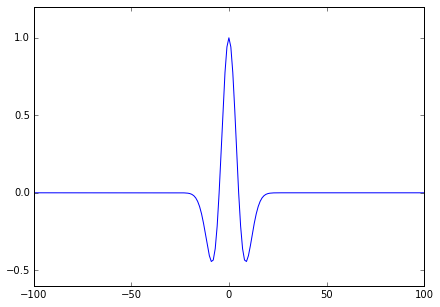In :
plot(t,fft.fftshift(abs(h_tf)))

Out:
[<matplotlib.lines.Line2D at 0x108607d10>]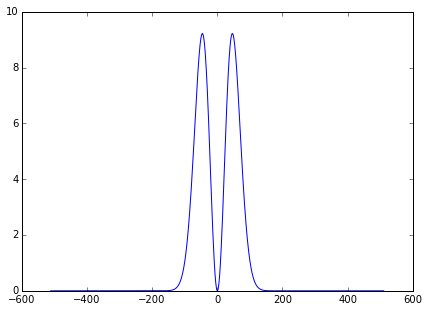We generate a synthetic sparse signal $x^\sharp$, with only a small number of nonzero coefficients.

In :
random.seed(80) # we set the seed of the random number generator for reproducibility purpose.

In :
s = round(N*.01) # number of nonzero elements of xsharp
sel = random.permutation(N)
sel = sel[0:s]   # indices of the nonzero elements of xsharp
xsharp = zeros(N)
xsharp[sel] = sign(randn(s)) * (1-0.3*rand(s))

In :
noiselevel = 0.2


Compute the measurements $y=A x^\sharp + w$ where $w$ is a realization of white Gaussian noise.

In :
y = opA(xsharp) + noiselevel * randn(N)

In :
figsize(14,5)
stem(sel,xsharp[sel])
xlim(0,N-1)
title('signal $x^\sharp$')

Out:
<matplotlib.text.Text at 0x108711090>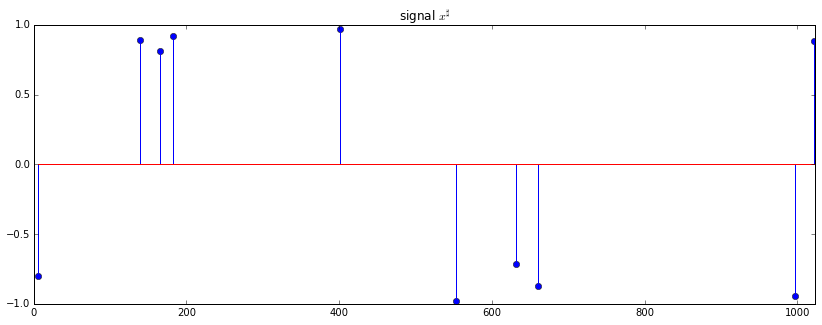In :
figsize(14,5)
plot(range(N),y)
xlim(0,N-1)
title('signal $y$')

Out:
<matplotlib.text.Text at 0x108a92110>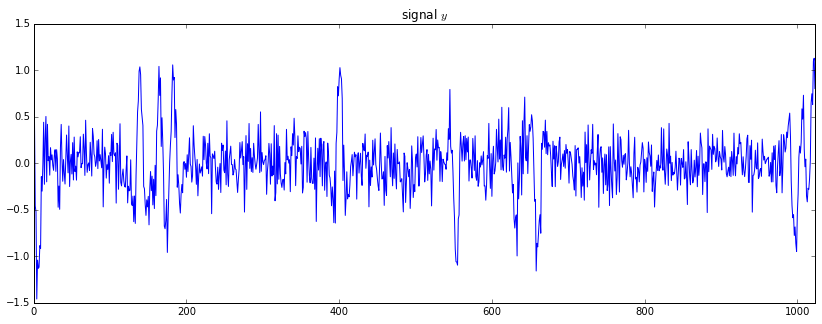## Deconvolution¶

We now implement the foward-backward algorithm to recover an estimate of the sparse signal

We define the regularization parameter $\la$.

In :
Lambda = 3


We define the proximity operator of $\ga g$.

In :
def prox_gamma_g (x, gamma, Lambda) :
return x - x/maximum(abs(x)/(Lambda*gamma),1) # soft-thresholding


We define the gradient operator of $f$. Note that $A^*=A$ because the filter $h$ is symmetric.

Exercise

Write the code of the function grad_f.

In :
grad_f = lambda x : # put your code here


We define the Lipschitz constant $\beta$ of $\nabla f$.

In :
beta = abs(fft.fft(h)).max()**2
beta

Out:
85.032184045506753

We define the stepsize $\ga$, which must be smaller than $2/\beta$.

In :
gamma = 1.9 / beta


We compute the solution of $\ell_1$ deconvolution (basis pursuit denoising). We keep track of the energy $E_k=f(x^{(k)})+g(x^{(k)})$.

Exercise

Write the code of the forward-backward iteration.

In :
nbiter = 2000
x = y
En_array = zeros(nbiter+1)
En_array = norm(opA(x) - y)**2/2 + Lambda*norm(x, ord=1)
for iter in range(nbiter):  # iter goes from 0 to nbiter-1
En_array[iter+1] = norm(opA(x) - y)**2/2 + Lambda*norm(x, ord=1)
x_restored = x


We display the result.

In :
fig, (subfig1,subfig2) = subplots(1,2,figsize=(16,7)) # one figure with two horizontal subfigures
subfig1.stem(xsharp)
subfig2.stem(x_restored)
subfig1.set_title('$x^\sharp$')
subfig2.set_title('$x_\mathrm{restored}$')

Out:
<matplotlib.text.Text at 0x1091d6e50>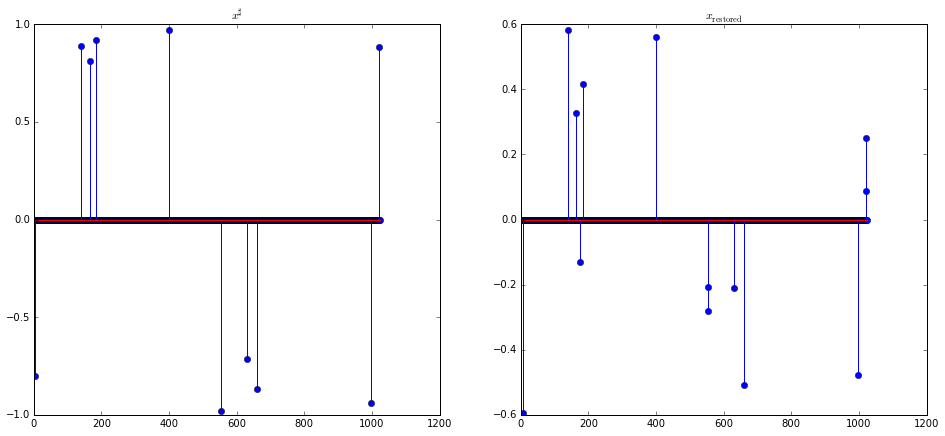We plot the relative error $(E_k-E^\star)/(E_0-E^\star)$ in log-scale with respect to $k$.

In :
plot(log10((En_array[0:1800]-En_array[-1])/(En_array-En_array[-1])))

Out:
[<matplotlib.lines.Line2D at 0x10cf32b90>]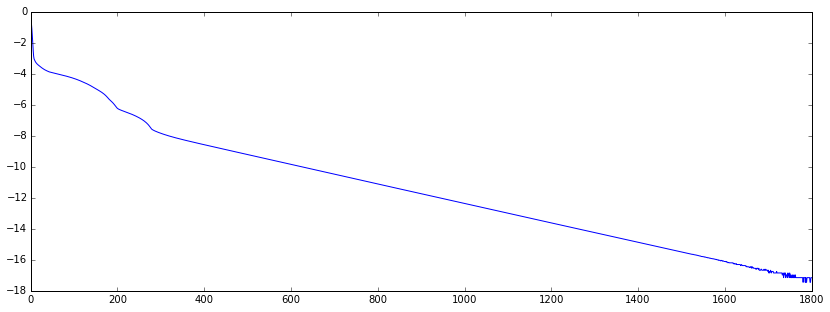## Over-relaxed Forward-Backward¶

It is possible to introduce a relaxation parameter $\rho$ with $0 < \rho < 1$. The over-relaxed foward-backward algorithm initializes $x^{(0)} \in \RR^N$, and then iterates, for $k=1,2,\ldots$ $$z^{(k)} = \text{prox}_{\ga g}\pa{ x^{(k-1)} - \ga \nabla f( x^{(k-1)} ) }.$$ $$x^{(k)} = z^{(k)} + \rho \pa{ z^{(k)} - x^{(k-1)} }$$

Let us assume $\gamma=1/\beta$. Convergence of the iterates $x^{(k)}$ and $z^{(k)}$ to a solution is guaranteed for $0 < \rho < 1/2$. The weaker property of convergence of $E(x^{(k)})$ to $E^\star$ is proved, when $1/2\leq \rho <1$.

Exercise

Write the code of the over-relaxed forward-backward iteration.

In :
gamma = 1/beta
nbiter = 1700
rho = 0.95
x = y
En_array_overrelaxed = zeros(nbiter+1)
En_array_overrelaxed = norm(opA(x) - y)**2/2 + Lambda*norm(x, ord=1)
for iter in range(nbiter):
En_array_overrelaxed[iter+1] = norm(opA(x) - y)**2/2 + Lambda*norm(x, ord=1)

In :
plot(log10((En_array[0:1800]-En_array[-1])/(En_array-En_array[-1])))
plot(log10((En_array_overrelaxed[0:1800]-En_array[-1])/(En_array-En_array[-1])))

Out:
[<matplotlib.lines.Line2D at 0x1085a78d0>]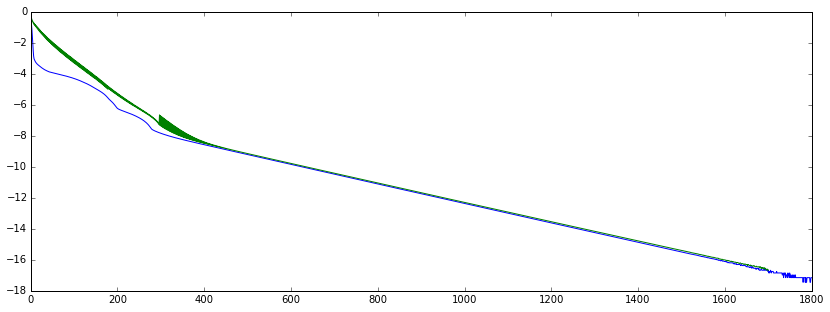As we see, in this example, over-relaxation does not bring any speedup, because $\gamma$ is lower than without over-relaxation. There are other setting parameters or other problems, for which over-relaxation does bring a significant speedup.

## FISTA-like Accelerated Forward-Backward Algorithm¶

We consider the FISTA algorithm introduced in:

A. Beck and M. Teboulle, "A Fast Iterative Shrinkage-Thresholding Algorithm for Linear Inverse Problems", SIAM Journal on Imaging Sciences, 2009.

More precisely, we consider a slightly modified version of FISTA, whose convergence is proved, see A. Chambolle and C. Dossal, "On the convergence of the iterates of "FISTA"", preprint, 2015.

Given an initial estimate $x^{(0)}$ of the solution and a parameter $a>2$, the algorithm sets $\gamma=1/\beta$, sets $z^{(0)}=x^{(0)} \in \RR^N$, and iterates, for $k=1,2,\ldots$ $$x^{(k)} = \text{prox}_{\ga g}\pa{ z^{(k-1)} - \ga \nabla f( z^{(k-1)} ) }.$$ $$\alpha_k=(k-1)/(k+a)$$ $$z^{(k)} = x^{(k)} + \alpha_k \pa{ x^{(k)} - x^{(k-1)} }$$

It is proved that the iterates $x^{(k)}$ converge to a solution $x^\star$ of the problem. Moreover, the optimal convergence rate for this class of problems is reached, namely $$E_k - E^\star = O(1/k^2),$$ whereas the convergence rate for the normal forward-backward is only $O(1/k)$.

Note the difference between the over-relaxed forward-backward and the accelerated forward-backward: the later is based on an inertia mechanism, of different nature than over-relaxation.

Exercise

Write the code of the accelerated forward-backward iteration.

In :
gamma = 1/beta
nbiter = 1700
a = 10
x = y
En_array_fista = zeros(nbiter+1)
En_array_fista = norm(opA(x) - y)**2/2 + Lambda*norm(x, ord=1)
for iter in range(nbiter):
En_array_fista[iter+1] = norm(opA(x) - y)**2/2 + Lambda*norm(x, ord=1)

In :
plot(log10((En_array[0:1800]-En_array[-1])/(En_array-En_array[-1])))
plot(log10((En_array_overrelaxed[0:1800]-En_array[-1])/(En_array-En_array[-1])))
plot(log10((En_array_fista[0:1800]-En_array[-1])/(En_array-En_array[-1])))

/Users/condatl/anaconda/lib/python2.7/site-packages/IPython/kernel/__main__.py:3: RuntimeWarning: divide by zero encountered in log10
app.launch_new_instance()
/Users/condatl/anaconda/lib/python2.7/site-packages/IPython/kernel/__main__.py:3: RuntimeWarning: invalid value encountered in log10
app.launch_new_instance()

Out:
[<matplotlib.lines.Line2D at 0x10d984c90>]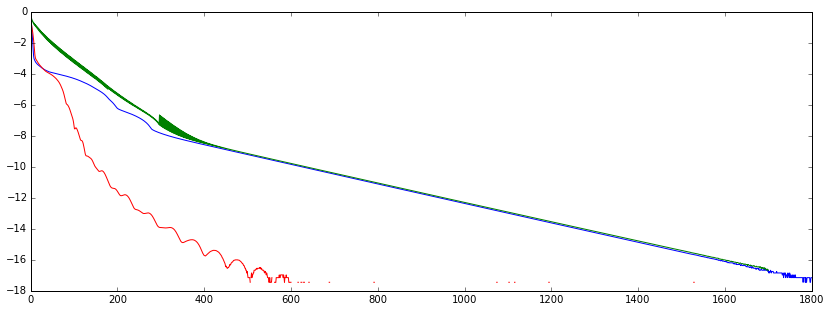Exercise

Try different values of a. You can try a=3,10,30,50,100.

We can note that the accelerated forward-backward is not monotonic: the cost function E is not decreasing along with the iterations and some oscillations are present.